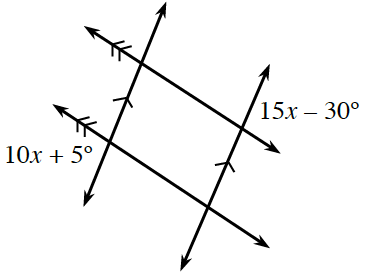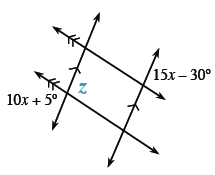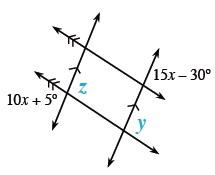### Home > GC > Chapter 2 > Lesson 2.1.5 > Problem2-51

2-51.Solve for $x$ in the diagram at right.

What is the relationship between angles $10x+5$ and $15x-30$?

The angle $10x+5$ is congruent to angle $z$.

Since angles $z$ and $y$ are corresponding angles on two parallel lines intersected by a transversal, they are also congruent.

The same rule applies to angle $y$ and the angle valued $15x-30$, so they are also congruent.Since $10x+5=z$, and $z=y=15x-30$, what can we conclude? Use your conclusion to solve for $x$.